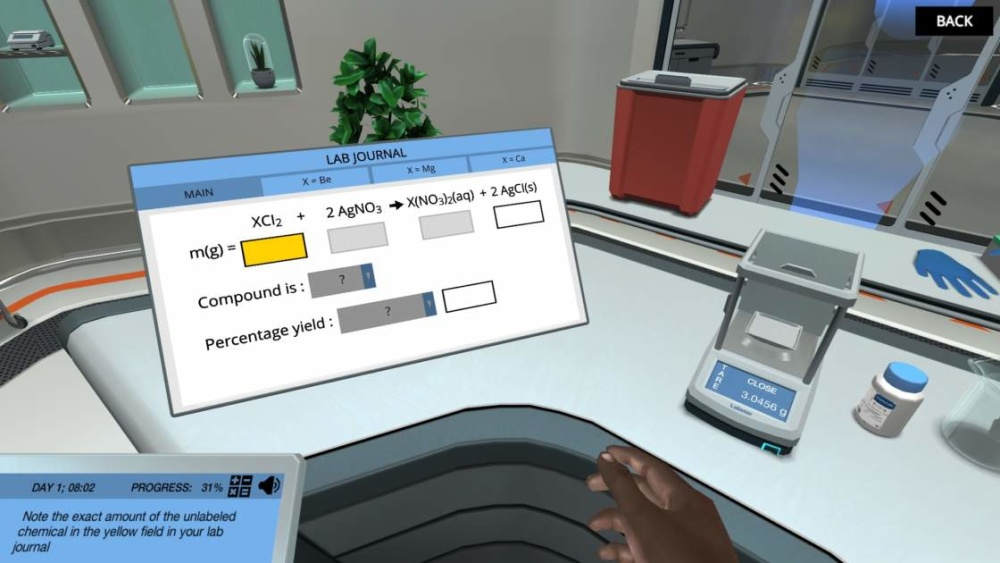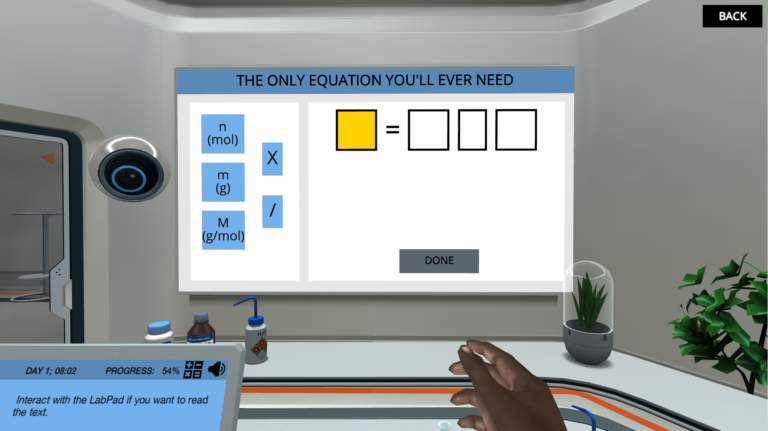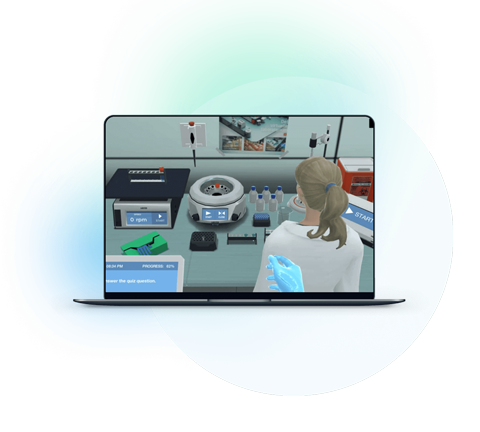Just as a delicious dish is made by adding the exact quantity of ingredients, a compound is formed when atoms of various elements react in correction proportion and quantity.

The branch of chemistry which involves the measurement of quantities and mass ratio of elements involved in a chemical reaction is called stoichiometry.

In stoichiometry, students learn about the concept of molecular mass, moles, and number molecules. They learn how to balance chemical equations and identify the compound with a gravimetric analysis (A technique to determine the amount of substance in a chemical reaction based on its mass).

Stoichiometry is considered the heart of chemistry with so many practical uses. The composition of all the chemical products we use in our daily lives, such as shampoos, cleaners, perfumes, soaps, and fertilizers is formed using stoichiometric calculations. Without stoichiometry, the chemical industry doesn’t exist.

Stoichiometry is one of the important topics that is usually taught in introductory chemistry courses. However, students consider it a bit tricky.Image from Labster's Stiochimoteric Calculations virtual lab.

Read on to learn reasons why students find it challenging and five ways to make it interesting for them.

## 3 Reasons Why students Stoichiometry Hard for Students

Stoichiometry is a dry and abstract concept. Following are three reasons why students consider it tough to comprehend:

### 1. Balancing Equations is Hard

One of the biggest challenges for students is balancing equations. This can be difficult because there are a lot of steps involved and students usually make many mistakes due to a lack of proper attention.  However, if you can break down the steps and make it simpler for students, they will likely succeed.

### 2. Molar Mass Calculations are Tough

Another difficult concept for students is molar mass calculations. To solve them, they need to be familiar with the molar masses of elements. Memorizing and applying them in the appropriate formulas is hard for them.

### 3. Students Don't See the Big Picture

Stoichiometry might be difficult for students because they often don't see the big picture. That is because they don't understand how all the concepts fit together and why they are being in the real world. If students can see the relevance of what they are learning, they will probably take more interest and feel motivated.

## 5 Ways to Make Stoichiometry Interesting for Students

Based on the three reasons why students find this topic hard, here is a list of five techniques you can use to make stoichiometry an approachable topic for students.

### 1. Explain Basic Terminologies and their Relationship

Stoichiometry is a complex topic. To make it easy to understand, you need to start with the very basic concepts. Such as you need to explain to them about molar mass, moles, and how the number of molecules is calculated.

Moles (n): Just as “dozen” is a unit of measurement, a mole is a unit to measure the amount of substance. One mole is defined as 6.022 x 1023atoms/molecules or 12 grams of carbon.

Molar Mass (M): Themass of one mole of an element is called its molar mass. For instance, the molar mass of carbon is12 grams per mole.

Molecular mass (m):  It isthe mass of one molecule of a particular element.

Relationship between n, M, and m

The three quantities are related such that n is the ratio of molecular mass (m) to molar mass (M).

n (mole) = m (g) / M (g/mol)

### 2. Mention Effective Methods to Balance a Chemical Equation

One of the main steps involved in stoichiometry is the balancing of chemical equations. The chemical equations mathematically describe the number of reactants and products involved in a chemical reaction. They tell us the exact ratio in which two or more reactants will combine to form products.

Most students have trouble balancing these equations. So, you can teach them two methods to balance these equations. One is the traditional method, and the second one is algebraic.

Balancing Chemical Equations with Traditional Method

Here’s a step-wise method that is commonly used to balance chemical equations:

Step 1: Write the chemical formula of reactants and products. For that, they must know exactly what compound is formed and what is its formula.  For instance, water is formed by the reaction of hydrogen (H2) and oxygen (O2).

Chemical Equation:  H2+O2🡪H2O

Step 2: Count no. of atoms on each element on either side of the equation.

Step 3: Add stoichiometric co-efficient to molecules whose atoms differ. For instance, we have two atoms of hydrogen on the reactant side but one atom on the product side. So, a co-efficient 2 is added to the right side.

Chemical Equation:  H2+O2🡪2H2O

Step 4: Keep repeating step 3 until the atoms on both sides become equal. For instance, in this case, when we added co-efficient 2 on the right side. The hydrogen atoms were imbalanced, so we need to add another coefficient, 2, on the left side with hydrogen.

Chemical Equation:  2H2+O2-🡪2H2O

Now, the equation is balanced with an equal amount of atoms of each element.

Balancing Chemical Equations with Algebraic Equations Method

Traditional balancing of equations is an iterative method, and this becomes hard when we encounter complex equations involving multiple reactants. In that case, we use a more advanced method that balances the chemical equation with algebraic equations.

Here’s a step-wise guide to the algebraic method of balancing equations:

Step 1: State the reactants and products of the chemical equation in their exact molecular formula. For instance, consider a reaction in which ethane (C2H6) reacts with oxygen (O2) to form carbon dioxide(CO2) and water(H2O).

C2H6 + O2  🡪 CO2+ H2O

Step 2: Add an algebraic variable to each reactant and product in the equation.

aC2H6 + bO2  🡪 cCO2+ dH2O

Step 3: Form an equation against atoms of each element. Since we have three elements and four variables, we can easily form three equations.

For Carbon: 2a = 1cFor Hydrogen: 6a = 2d; d=3a

For Oxygen: 2b = 2c+d;

Step 4: Solve the equation for variables. The equation can have multiple solutions. However, if we specify the value of one variable i.e a=2, we get a whole number value of all other coefficients.

When, a=2, c=4, d=6, and b =7.

Step 5: Write the final equation.

2C2H6 + 7O2  🡪 4CO2+ 6H2OImage from Labster's Avogadro’s Number and molecular calculations.

### 3. Relate it to the Real World: Cite Applications

Chemistry is a dry field and these complex equations seem abstract to students. Students cannot figure out how stoichiometry is used in the real world. Hence, they don’t realize its importance and aren’t energized to learn about it.

Therefore, it becomes necessary on your part to explain the real-life applications to them. When they realize how stoichiometry is being used practically; they would get excited and interested in learning.

Stoichiometry is used in various fields and applications. Here are some interesting applications of stoichiometric calculations:

Product Identification: Stoichiometry is used in product identification. It helps to determine the exact amount and purity of a product. For instance, when we know the elemental composition of a particular steel, we can use stoichiometric calculations to identify its grade.

Cooking: Stoichiometry also has a role in cooking. For instance, when we know how many people will be eating and what quantity of food they want to eat, we can use stoichiometric calculations to prepare that food.

Pharmaceutical Industry: In the pharmaceutical industry, it is used to create new medicines/drugs and identify their chemical composition.

FMCGs: In the FMCG industry, it is used in product formulations. For instance, when we have to prepare 1,000 liters of shampoo, we can use stoichiometric calculations to find out the exact amount of each ingredient that needs to be added.

Agriculture: Stoichiometry also has applications in the agriculture field. It helps to determine the right amount of fertilizer that needs to be added to the soil to get the desired results.

### 4. Take them on a Field Tour

Apart from mentioning the applications, it would be a great idea if you could arrange a field tour for them. This could be a trip to a local chemical factory or company that uses chemical reactions on a large scale. Seeing how the concepts they learn in class are applied in the real world can be very motivating for students.

### 5. Use Virtual Lab Simulation

Teaching something dry like chemical equations with simulations is another wonderful technique. You can explain the tough and boring equations to students with colorful animations and visuals.

In regards to simulations, Labster can help you greatly. Students can easily learn complex and abstract concepts through our gamified and story-based environment.

For Stoichiometry, we have designed a couple of simulations. For instance, in one simulation on Avogadro’s Number and molecular calculations, students get familiarized with the concept of moles and molecules. Then, in an advanced simulation on Stiochimoteric Calculations, they identify an unknown compound using gravimetric analysis.